# JavaScript纯符号输出文本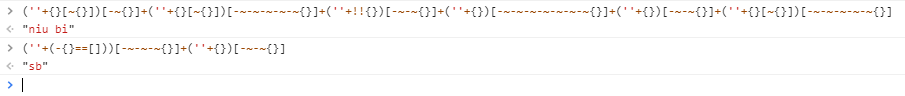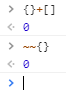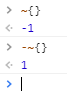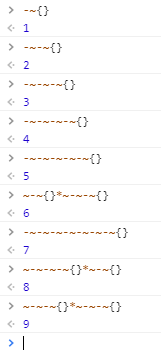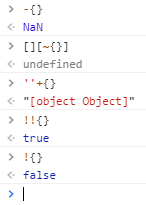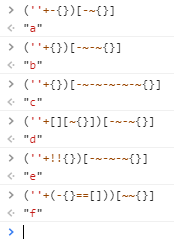```1 2 3 4 5 6 7 8 9 10 11 12 13 14 15 16 17 18 19 20 21 22 23 24 25 26 27 28 29 30 31 32 33 34 ~~{} // 0 ~{} // -1 -~{} // 1 ~-~{} // -2 -~-~{} // 2 ~-~-~{} // -3 -~-~-~{} // 3 ~-~-~-~{} // -4 -~-~-~-~{} // 4 -~-~-~-~-~{} // 5 ~-~{}*~-~-~{} // 6 -~-~-~-~-~-~-~{} // 7 ~-~-~-~{}*~-~{} // 8 ~-~-~{}*~-~-~{} // 9 (''+-{})[-~{}] // a (''+{})[-~-~{}] // b (''+{})[-~-~-~-~-~{}] // c (''+[][~{}])[-~-~{}] // d (''+!!{})[-~-~-~{}] // e (''+(-{}==[]))[~~{}] // f // g h 无法展示 (''+[][~{}])[-~-~-~-~-~{}] // i (''+{})[-~-~-~{}] // j // k l m 无法展示 (''+[][~{}])[-~{}] // n (''+{})[-~{}] // o // p q 无法展示 (''+!!{})[-~{}] // r (''+(-{}==[]))[-~-~-~{}] // s (''+!!{})[~~{}] // t (''+!!{})[-~-~{}] // u // v w x y z 无法展示 (''+-{})[~~{}] // N (''+{})[~-~-~-~{}*~-~{}] // O```